The axisymmetric equations of linear elasticity

The aim of this tutorial is to demonstrate the solution of the axisymmetric equations of linear elasticity in cylindrical polar coordinates.

 Acknowledgement: This implementation of the equations and the documentation were developed jointly with Matthew Russell with financial support to Chris Bertram from the Chiari and Syringomyelia Foundation.

# Theory

Consider a three-dimensional, axisymmetric body (of density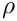, Young's modulus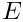, and Poisson's ratio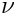), occupying the region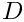whose boundary is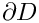. Using cylindrical coordinates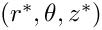, the equations of linear elasticity can be written as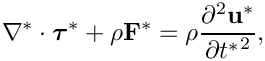where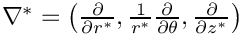,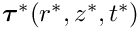is the stress tensor,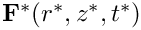is the body force and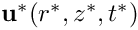is the displacement field.

Note that, despite the fact that none of the above physical quantities depend on the azimuthal angle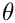, each can have a non-zerocomponent. Also note that variables written with a superscript asterisk are dimensional, and their non-dimensional counterparts will be written without an asterisk. (The coordinateis, by definition, non-dimensional, so it will always be written without an asterisk.)

A boundary traction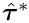and boundary displacement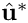are imposed along the boundaries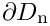and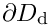respectively, where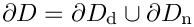so that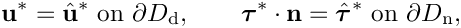where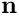is the outer unit normal vector.

The constitutive equations relating the stresses to the displacements are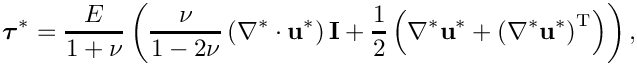where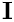is the identity tensor and superscript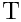denotes the transpose. In cylindrical coordinates, the matrix representation of the tensor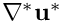is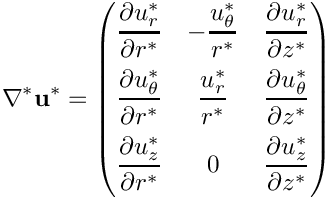and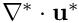is equal to the trace of this matrix.

We non-dimensionalise the equations, using a problem specific reference length,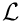, and a timescale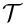, and use Young's modulus to non-dimensionalise the body force and the stress tensor: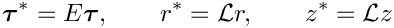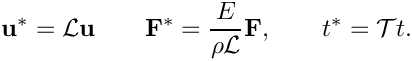The non-dimensional form of the axisymmetric linear elasticity equations is then given by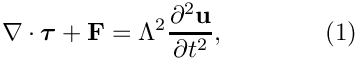where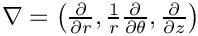,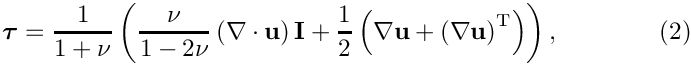and the non-dimensional parameter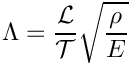is the ratio of the elastic body's intrinsic timescale,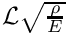, to the problem-specific timescale,, that we use for time-dependent problems. The boundary conditions are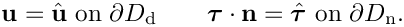We must also specify initial conditions: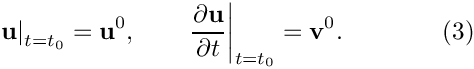# Implementation

Within oomph-lib, the non-dimensional version of the axisymmetric linear elasticity equations (1) combined with the constitutive equations (2) are implemented in the AxisymmetricLinearElasticityEquations class. This class implements the equations in a way which is general with respect to the specific element geometry. To obtain a fully functioning element class, we must combine the equations class with a specific geometric element class, as discussed in the (Not-So-)Quick Guide. For example, we will combine the AxisymmetricLinearElasticityEquations class with QElement<2,3> elements, which are 9-node quadrilateral elements, in our example problem. As usual, the mapping between local and global (Eulerian) coordinates within an element is given by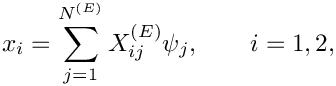where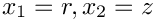;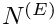is the number of nodes in the element.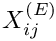is the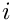-th global (Eulerian) coordinate of the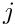-th node in the element and the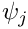are the element's shape functions, which are specific to each type of geometric element.

We store the three components of the displacement vector as nodal data in the order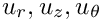and use the shape functions to interpolate the displacements as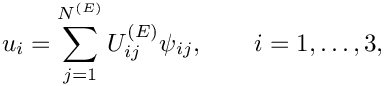where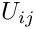is the-th displacement component at the-th node in the element, i.e.,.

The solution of time dependent problems requires the specification of a TimeStepper that is capable of approximating second time derivatives. In the example problem below we use the Newmark timestepper.

# The test problem

As a test problem we consider forced oscillations of the circular cylinder shown in the sketch below: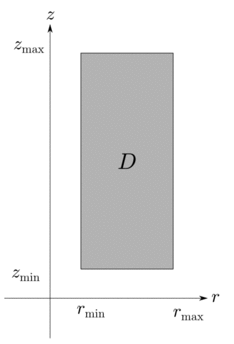Azimuthal cross-section of the geometry.

It is difficult to find non-trivial exact solutions of the governing equations (1), (2), so we manufacture a time-harmonic solution: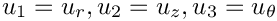This is an exact solution if we set the body force to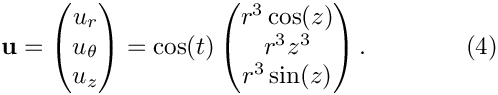where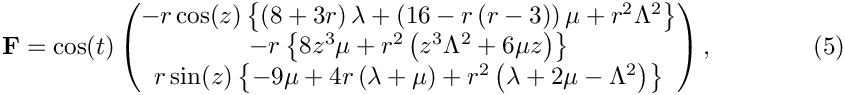and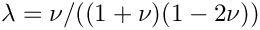are the nondimensional Lamé parameters. We impose the displacement along the boundaries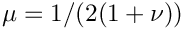according to (4), and impose the traction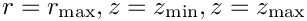along the boundary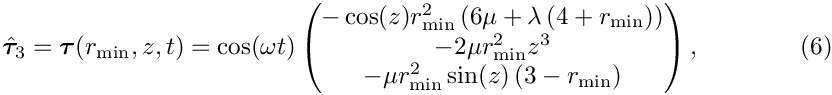.

The problem we are solving then consists of equations (1), (2) along with the body force, (5) and boundary traction (6). The initial conditions for the problem are the exact displacement, velocity (and acceleration; see below) according to the solution (4).

# Results

The animation below shows the time dependent deformation of the cylinder in the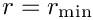-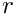plane, while the colour contours indicate the azimuthal displacement component. The animation is for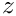, since the time scale is nondimensionalised on the reciprocal of the angular frequency of the oscillations.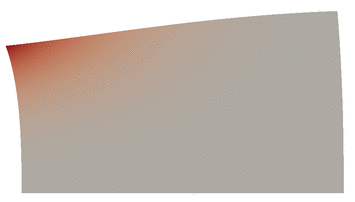Animation (HTML only) of the resulting displacement field.

The next three figures show plots of the radial, axial and azimuthal displacements as functions of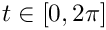at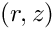. Note the excellent agreement between the numerical and exact solutions.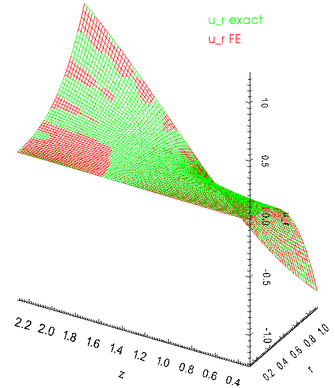Comparison between exact and FE solutions for the r-component of displacement at t = 2.64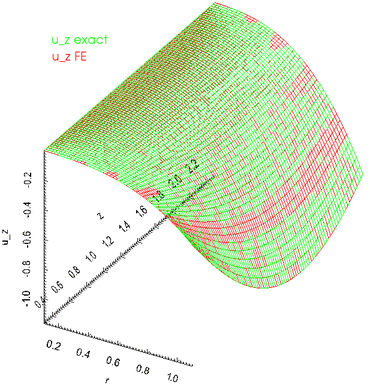Comparison between exact and FE solutions for the z-component of displacement at t = 2.64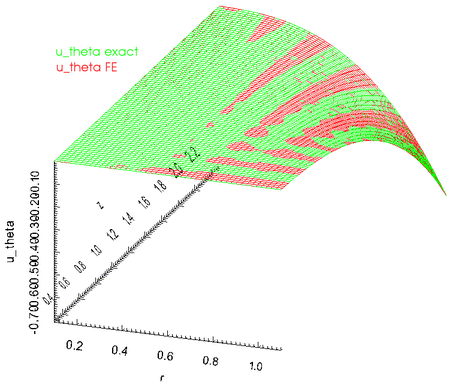Comparison between exact and FE solutions for the theta-component of displacement at t = 2.64

# Global parameters and functions

As usual, we define all non-dimensional parameters in a namespace. In this namespace, we also define the body force, the traction to be applied on the boundary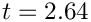, and the exact solution. Note that, consistent with the enumeration of the unknowns, discussed above, the order of the components in the functions that specify the body force and the surface traction is.

//===start_of_Global_Parameters_namespace===============================
/// Namespace for global parameters
//======================================================================
{
/// Define Poisson's ratio Nu
double Nu = 0.3;
/// Define the non-dimensional Young's modulus
double E = 1.0;
/// Lame parameters
double Lambda = E*Nu/(1.0+Nu)/(1.0-2.0*Nu);
double Mu = E/2.0/(1.0+Nu);
/// Square of the frequency of the time dependence
double Omega_sq = 0.5;
/// Number of elements in r-direction
unsigned Nr = 5;
/// Number of elements in z-direction
unsigned Nz = 10;
/// Length of domain in r direction
double Lr = 1.0;
/// Length of domain in z-direction
double Lz = 2.0;
/// Set up min r coordinate
double Rmin = 0.1;
/// Set up min z coordinate
double Zmin = 0.3;
/// Set up max r coordinate
double Rmax = Rmin+Lr;
/// Set up max z coordinate
double Zmax = Zmin+Lz;
/// The traction function at r=Rmin: (t_r, t_z, t_theta)
void boundary_traction(const double &time,
const Vector<double> &x,
const Vector<double> &n,
Vector<double> &result)
{
result = cos(time)*(-6.0*pow(x,2)*Mu*cos(x)-
Lambda*(4.0*pow(x,2)+pow(x,3))*cos(x));
result = cos(time)*(-Mu*(3.0*pow(x,2)-pow(x,3))*sin(x));
result = cos(time)*(-Mu*pow(x,2)*(2*pow(x,3)));
}
/// The body force function; returns vector of doubles
/// in the order (b_r, b_z, b_theta)
void body_force(const double &time,
const Vector<double> &x,
Vector<double> &result)
{
result = cos(time)*(
x*(-cos(x)*
(Lambda*(8.0+3.0*x)-
Mu*(-16.0+x*(x-3.0))+pow(x,2)*Omega_sq)));
result = cos(time)*(
x*sin(x)*(Mu*(-9.0)+
4.0*x*(Lambda+Mu)+pow(x,2)*
(Lambda+2.0*Mu-Omega_sq)));
result = cos(time)*(
-x*(8.0*Mu*pow(x,3)+pow(x,2)*(pow(x,3)*Omega_sq+6.0*Mu*x)));
} // end of body force
Namespace for global parameters.
Definition: cylinder.cc:44
double Rmax
Set up max r coordinate.
Definition: cylinder.cc:77
double Zmin
Set up min z coordinate.
Definition: cylinder.cc:74
unsigned Nz
Number of elements in z-direction.
Definition: cylinder.cc:62
double Nu
Define Poisson's ratio Nu.
Definition: cylinder.cc:46
double Lz
Length of domain in z-direction.
Definition: cylinder.cc:68
double Zmax
Set up max z coordinate.
Definition: cylinder.cc:80
double Lr
Length of domain in r direction.
Definition: cylinder.cc:65
void boundary_traction(const double &time, const Vector< double > &x, const Vector< double > &n, Vector< double > &result)
The traction function at r=Rmin: (t_r, t_z, t_theta)
Definition: cylinder.cc:83
void body_force(const double &time, const Vector< double > &x, Vector< double > &result)
The body force function; returns vector of doubles in the order (b_r, b_z, b_theta)
Definition: cylinder.cc:96
double E
Define the non-dimensional Young's modulus.
Definition: cylinder.cc:49
double Rmin
Set up min r coordinate.
Definition: cylinder.cc:71
double Lambda
Lame parameters.
Definition: cylinder.cc:52
unsigned Nr
Number of elements in r-direction.
Definition: cylinder.cc:59
double Omega_sq
Square of the frequency of the time dependence.
Definition: cylinder.cc:56

In addition, the namespace includes the necessary machinery for providing the time dependent equations with their initial data from the exact solution. There are 9 functions, one for each of the components of displacement, velocity and acceleration, and a helper function. For brevity, we list only one of these functions; the others are similar.

/// Calculate the time dependent form of the r-component of displacement
double u_r(const double &time, const Vector<double> &x)
{
Vector<double> displ(3);
return cos(time)*displ;
} // end_of_u_r
void exact_solution_th(const Vector< double > &x, Vector< double > &u)
Helper function - spatial components of the exact solution in a vector. This is necessary because we ...
Definition: cylinder.cc:116
double u_r(const double &time, const Vector< double > &x)
Calculate the time dependent form of the r-component of displacement.
Definition: cylinder.cc:130

# The driver code

We start by creating a DocInfo object that will be used to output the solution, and then build the problem.

//===start_of_main======================================================
/// Driver code
//======================================================================
int main(int argc, char* argv[])
{
// Store command line arguments
CommandLineArgs::setup(argc,argv);
// Define possible command line arguments and parse the ones that
// were actually specified
// Validation?
CommandLineArgs::specify_command_line_flag("--validation");
// Parse command line
CommandLineArgs::parse_and_assign();
// Doc what has actually been specified on the command line
CommandLineArgs::doc_specified_flags();
// Set up doc info
DocInfo doc_info;
// Set output directory
doc_info.set_directory("RESLT");
// Time dependent problem instance
<QAxisymmetricLinearElasticityElement<3>, Newmark<1> > problem;
Class to validate time harmonic linear elasticity (Fourier decomposed)
Definition: cylinder.cc:228
int main(int argc, char *argv[])
Driver code.
Definition: cylinder.cc:714

Next we set up a timestepper and assign the initial conditions.

// Set the initial time to t=0
problem.time_pt()->time()=0.0;
// Set and initialise timestep
double dt=0;
// If we're validating, use a larger timestep so that we can do fewer steps
// before reaching interesting behaviour
if(CommandLineArgs::command_line_flag_has_been_set("--validation"))
{
dt=0.1;
}
else // Otherwise use a small timestep
{
dt=0.01;
}
problem.time_pt()->initialise_dt(dt);
// Set the initial conditions
problem.set_initial_conditions();
// Doc the initial conditions and increment the doc_info number
problem.doc_solution(doc_info);
doc_info.number()++;

We calculate the number of timesteps to perform - if we are validating, just do small number of timesteps; otherwise do a full period the time-harmonic oscillation.

// Find the number of timesteps to perform
unsigned nstep=0;
// If we're validating, only do a few timesteps; otherwise do a whole period
if(CommandLineArgs::command_line_flag_has_been_set("--validation"))
{
nstep=5;
}
else // Otherwise calculate based on timestep
{
// Solve for one full period
double t_max=2*MathematicalConstants::Pi;
nstep=unsigned(t_max/dt);
} //end_of_calculate_number_of_timesteps

Finally we perform a time dependent simulation.

// Do the timestepping
for(unsigned istep=0;istep<nstep;istep++)
{
// Solve for this timestep
// Doc the solution and increment doc_info number
problem.doc_solution(doc_info);
doc_info.number()++;
}
} // end_of_main

# The problem class

The problem class is very simple, and similarly to other problems with Neumann conditions, there are separate meshes for the "bulk" elements and the "face" elements that apply the traction boundary conditions. The function assign_traction_elements() attaches the traction elements to the appropriate bulk elements.

//===start_of_problem_class=============================================
/// Class to validate time harmonic linear elasticity (Fourier
/// decomposed)
//======================================================================
template<class ELEMENT, class TIMESTEPPER>
{
public:
/// Constructor: Pass number of elements in r and z directions,
/// boundary locations and whether we are doing an impulsive start or not
/// Update before solve is empty
/// Update after solve is empty
/// Actions before implicit timestep
{
// Just need to update the boundary conditions
}
/// Set the initial conditions, either for an impulsive start or
/// with history values for the time stepper
/// Set the boundary conditions
/// Doc the solution
void doc_solution(DocInfo& doc_info);
private:
/// Allocate traction elements on the bottom surface
/// Pointer to the bulk mesh
Mesh* Bulk_mesh_pt;
/// Pointer to the mesh of traction elements
}; // end_of_problem_class
void actions_before_newton_solve()
Update before solve is empty.
Definition: cylinder.cc:236
void doc_solution(DocInfo &doc_info)
Doc the solution.
Definition: cylinder.cc:664
Mesh * Bulk_mesh_pt
Pointer to the bulk mesh.
Definition: cylinder.cc:264
Mesh * Surface_mesh_pt
Pointer to the mesh of traction elements.
Definition: cylinder.cc:267
void set_initial_conditions()
Set the initial conditions, either for an impulsive start or with history values for the time stepper...
Definition: cylinder.cc:416
void actions_before_implicit_timestep()
Actions before implicit timestep.
Definition: cylinder.cc:242
AxisymmetricLinearElasticityProblem()
Constructor: Pass number of elements in r and z directions, boundary locations and whether we are doi...
Definition: cylinder.cc:277
void assign_traction_elements()
Allocate traction elements on the bottom surface.
Definition: cylinder.cc:388
void set_boundary_conditions()
Set the boundary conditions.
Definition: cylinder.cc:574
void actions_after_newton_solve()
Update after solve is empty.
Definition: cylinder.cc:239

# The problem constructor

The problem constructor creates the mesh objects (which in turn create the elements), pins the appropriate boundary nodes and assigns the boundary conditions according to the functions defined in the Global_Parameters namespace.

//===start_of_constructor=============================================
/// Problem constructor: Pass number of elements in coordinate
/// directions and size of domain.
//====================================================================
template<class ELEMENT, class TIMESTEPPER>
{
//Allocate the timestepper
//Now create the mesh
time_stepper_pt());
//Create the surface mesh of traction elements
Surface_mesh_pt=new Mesh;
assign_traction_elements();
//Set the boundary conditions
set_boundary_conditions();

Then the physical parameters are set for each element in the bulk mesh.

// Complete the problem setup to make the elements fully functional
// Loop over the elements
unsigned n_el = Bulk_mesh_pt->nelement();
for(unsigned e=0;e<n_el;e++)
{
// Cast to a bulk element
ELEMENT *el_pt = dynamic_cast<ELEMENT*>(Bulk_mesh_pt->element_pt(e));
// Set the body force
el_pt->body_force_fct_pt() = &Global_Parameters::body_force;
// Set the pointer to Poisson's ratio
el_pt->nu_pt() = &Global_Parameters::Nu;
// Set the pointer to non-dim Young's modulus
el_pt->youngs_modulus_pt() = &Global_Parameters::E;
// Set the pointer to the Lambda parameter
el_pt->lambda_sq_pt() = &Global_Parameters::Omega_sq;
}// end_loop_over_elements

We then loop over the traction elements and set the applied traction.

// Loop over the traction elements
unsigned n_traction = Surface_mesh_pt->nelement();
for(unsigned e=0;e<n_traction;e++)
{
// Cast to a surface element
AxisymmetricLinearElasticityTractionElement<ELEMENT>*
el_pt =
dynamic_cast<AxisymmetricLinearElasticityTractionElement
<ELEMENT>* >(Surface_mesh_pt->element_pt(e));
// Set the applied traction
el_pt->traction_fct_pt() = &Global_Parameters::boundary_traction;
}// end_loop_over_traction_elements

Finally, we add the two meshes as sub-meshes, build a global mesh from these and assign the equation numbers.

// Add the submeshes to the problem
// Now build the global mesh
build_global_mesh();
// Assign equation numbers
cout << assign_eqn_numbers() << " equations assigned" << std::endl;
} // end_of_constructor

# The traction elements

We create the face elements that apply the traction to the boundary.

//===start_of_traction===============================================
/// Make traction elements along the boundary r=Rmin
//===================================================================
template<class ELEMENT, class TIMESTEPPER>
{
unsigned bound, n_neigh;
// How many bulk elements are next to boundary 3
bound=3;
n_neigh = Bulk_mesh_pt->nboundary_element(bound);
// Now loop over bulk elements and create the face elements
for(unsigned n=0;n<n_neigh;n++)
{
// Create the face element
FiniteElement *traction_element_pt
= new AxisymmetricLinearElasticityTractionElement<ELEMENT>
(Bulk_mesh_pt->boundary_element_pt(bound,n),
Bulk_mesh_pt->face_index_at_boundary(bound,n));
}
} // end of assign_traction_elements

# Initial data

The time integration in this problem is performed using the Newmark scheme which, in addition to the standard initial conditions (3), requires an initial value for the acceleration. Since we will be solving a test case in which the exact solution is known, we can use the exact solution to provide the complete set of initial data required. For the details of the Newmark scheme, see the tutorial on the linear wave equation.

If we're doing an impulsive start, set the displacement, velocity and acceleration to zero, and fill in the time history to be consistent with this.

//===start_of_set_initial_conditions=================================
/// Set the initial conditions (history values)
//===================================================================
template<class ELEMENT, class TIMESTEPPER>
{
// Upcast the timestepper to the specific type we have
TIMESTEPPER* timestepper_pt =
dynamic_cast<TIMESTEPPER*>(time_stepper_pt());
// By default do a non-impulsive start and provide initial conditions
bool impulsive_start=false;
if(impulsive_start)
{
// Number of nodes in the bulk mesh
unsigned n_node = Bulk_mesh_pt->nnode();
// Loop over all nodes in the bulk mesh
for(unsigned inod=0;inod<n_node;inod++)
{
// Pointer to node
Node* nod_pt = Bulk_mesh_pt->node_pt(inod);
// Get nodal coordinates
Vector<double> x(2);
x = nod_pt->x(0);
x = nod_pt->x(1);
// Assign zero solution at t=0
nod_pt->set_value(0,0);
nod_pt->set_value(1,0);
nod_pt->set_value(2,0);
// Set the impulsive initial values in the timestepper
timestepper_pt->assign_initial_values_impulsive(nod_pt);
}
} // end_of_impulsive_start

If we are not doing an impulsive start, we must provide the timestepper with time history values for the displacement, velocity and acceleration. Each component of the these vectors is represented by a function pointer, and in this case, the function pointers return values based on the exact solution.

else // Smooth start
{
// Storage for pointers to the functions defining the displacement,
// velocity and acceleration components
Vector<typename TIMESTEPPER::NodeInitialConditionFctPt>
initial_value_fct(3);
Vector<typename TIMESTEPPER::NodeInitialConditionFctPt>
initial_veloc_fct(3);
Vector<typename TIMESTEPPER::NodeInitialConditionFctPt>
initial_accel_fct(3);
// Set the displacement function pointers
initial_value_fct=&Global_Parameters::u_r;
initial_value_fct=&Global_Parameters::u_z;
initial_value_fct=&Global_Parameters::u_theta;
// Set the velocity function pointers
initial_veloc_fct=&Global_Parameters::d_u_r_dt;
initial_veloc_fct=&Global_Parameters::d_u_z_dt;
initial_veloc_fct=&Global_Parameters::d_u_theta_dt;
// Set the acceleration function pointers
initial_accel_fct=&Global_Parameters::d2_u_r_dt2;
initial_accel_fct=&Global_Parameters::d2_u_z_dt2;
initial_accel_fct=&Global_Parameters::d2_u_theta_dt2;
double d2_u_z_dt2(const double &time, const Vector< double > &x)
Calculate the time dependent form of the z-component of acceleration.
Definition: cylinder.cc:186
double d2_u_r_dt2(const double &time, const Vector< double > &x)
Calculate the time dependent form of the r-component of acceleration.
Definition: cylinder.cc:178
double d_u_theta_dt(const double &time, const Vector< double > &x)
Calculate the time dependent form of the theta-component of velocity.
Definition: cylinder.cc:170
double d2_u_theta_dt2(const double &time, const Vector< double > &x)
Calculate the time dependent form of the theta-component of acceleration.
Definition: cylinder.cc:194
double d_u_r_dt(const double &time, const Vector< double > &x)
Calculate the time dependent form of the r-component of velocity.
Definition: cylinder.cc:154
double d_u_z_dt(const double &time, const Vector< double > &x)
Calculate the time dependent form of the z-component of velocity.
Definition: cylinder.cc:162
double u_z(const double &time, const Vector< double > &x)
Calculate the time dependent form of the z-component of displacement.
Definition: cylinder.cc:138
double u_theta(const double &time, const Vector< double > &x)
Calculate the time dependent form of the theta-component of displacement.
Definition: cylinder.cc:146

Then we loop over all nodes in the bulk mesh and set the initial data values from the exact solution.

// Number of nodes in the bulk mesh
unsigned n_node = Bulk_mesh_pt->nnode();
// Loop over all nodes in bulk mesh
for(unsigned inod=0;inod<n_node;inod++)
{
// Pointer to node
Node* nod_pt = Bulk_mesh_pt->node_pt(inod);
// Assign the history values
timestepper_pt->assign_initial_data_values(nod_pt,
initial_value_fct,
initial_veloc_fct,
initial_accel_fct);
} // end_of_loop_over_nodes

# Post-processing

This member function documents the computed solution to file and calculates the error between the computed solution and the exact solution.

//==start_of_doc_solution=================================================
/// Doc the solution
//========================================================================
template<class ELEMENT, class TIMESTEPPER>
doc_solution(DocInfo& doc_info)
{
ofstream some_file;
char filename;
// Number of plot points
unsigned npts=10;
// Output solution
sprintf(filename,"%s/soln%i.dat",doc_info.directory().c_str(),
doc_info.number());
some_file.open(filename);
Bulk_mesh_pt->output(some_file,npts);
some_file.close();
// Output exact solution
sprintf(filename,"%s/exact_soln%i.dat",doc_info.directory().c_str(),
doc_info.number());
some_file.open(filename);
Bulk_mesh_pt->output_fct(some_file,npts,time_pt()->time(),
some_file.close();
// Doc error
double error=0.0;
double norm=0.0;
sprintf(filename,"%s/error%i.dat",doc_info.directory().c_str(),
doc_info.number());
some_file.open(filename);
Bulk_mesh_pt->compute_error(some_file,
time_pt()->time(),
error,norm);
some_file.close();
// Doc error norm:
cout << "\nNorm of error: " << sqrt(error) << std::endl;
cout << "Norm of solution: " << sqrt(norm) << std::endl << std::endl;
cout << std::endl;
} // end_of_doc_solution
void exact_solution(const double &time, const Vector< double > &x, Vector< double > &u)
The exact solution in a vector: 0: u_r, 1: u_z, 2: u_theta and their 1st and 2nd derivs.
Definition: cylinder.cc:203

• Given that we non-dimensionalised all stresses on Young's modulus it seems odd that we provide the option to specify a non-dimensional Young's modulus via the member function AxisymmetricLinearElasticity::youngs_modulus_pt(). The explanation for this is that this function specifies the ratio of the material's actual Young's modulus to the Young's modulus used in the non-dimensionalisation of the equations. The capability to specify such ratios is important in problems where the elastic body is made of multiple materials with different constitutive properties. If the body is made of a single, homogeneous material, the specification of the non-dimensional Young's modulus is not required – it defaults to 1.0.

# Exercises

• Try setting the boolean flag impulsive_start to true in the AxisymmetricLinearElasticityProblem::set_initial_conditions function and compare the system's evolution to that obtained when a "smooth" start from the exact solution is performed.
• Omit the specification of the Young's modulus and verify that the default value gives the same solution.
• Confirm that the assignment of the history values for the Newmark timestepper in AxisymmetricLinearElasticityProblem::set_initial_conditions sets the correct initial values for the displacement, velocity and acceleration. (Hint: the relevant code is already contained in the driver code, but was omitted in the code listings shown above.)